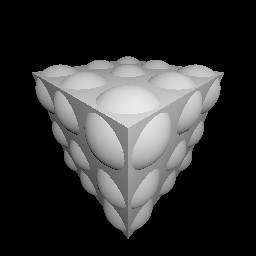### 6Transformation

TODO: exposition about linear transformations and affine transformations

#### 6.1Basic Transformation Data Types

 type
 predicate
The type and predicate for linear transformations.

 procedure(linear dx dy dz) → Linear dx : Dir dy : Dir dz : Dir
Converts three axes into a linear transformation.

 procedure(linear-x-axis t) → Dir t : Linear
 procedure(linear-y-axis t) → Dir t : Linear
 procedure(linear-z-axis t) → Dir t : Linear
Return the axes of t separately; i.e.

(match-define (linear dx dy dz) t)

is equivalent to
 (define dx (linear-x-axis t)) (define dy (linear-y-axis t)) (define dz (linear-z-axis t))

 value
The identity linear transformation: each axis is a coordinate axis.

Example:
 > identity-linear (linear +x +y +z)

 procedure(linear-compose t ...) → Linear t : Linear
Composes any number of linear transformations. Applying the result applies each t once, in reverse order (just like compose).

 procedure t : Linear
Returns the inverse of the transformation t. Because Linear instances store their inverses, this operation is cheap.

If t isn’t invertible, linear-inverse raises an error. See linear-singular?.

 procedure t : Linear
Returns #t when (linear-inverse t) would raise an error.

 type
 predicate
The type and predicate for parallel-line-preserving transformations.

 procedure(affine dx dy dz p) → Affine dx : Dir dy : Dir dz : Dir p : Pos
Converts three axes and an origin into an affine transformation.

 procedure(affine-x-axis t) → Dir t : Affine
 procedure(affine-y-axis t) → Dir t : Affine
 procedure(affine-z-axis t) → Dir t : Affine
 procedure(affine-origin t) → Pos t : Affine
Return the axes and origin of t separately; i.e.

(match-define (affine dx dy dz p) t)

is equivalent to
 (define dx (affine-x-axis t)) (define dy (affine-y-axis t)) (define dz (affine-z-axis t)) (define p  (affine-origin t))

 value
The identity affine transformation: each axis is a coordinate axis, and its origin is origin.

Examples:
 > identity-affine (linear +x +y +z) > (affine-origin identity-affine) origin

 procedure(affine-compose t ...) → Affine t : Affine
Composes any number of affine transformations. Applying the result applies each t once, in reverse order (just like compose).

 procedure t : Affine
Returns the inverse of the transformation t. Because Affine instances store their inverses, this operation is cheap.

If t isn’t invertible, affine-inverse raises an error. See affine-singular?.

 procedure t : Affine
Returns #t when (affine-inverse t) would raise an error.

 procedure t : Linear
 procedure t : Affine
Return #t when t preserves orientation, or handedness. An inconsistent transformation turns clockwise-oriented shapes or directions counterclockwise, and vice-versa.

Some 3D engines are sensitive to consistency: they will render shapes inside-out or turn normals the wrong direction when inconsistent transformations are applied. Pict3D’s rendering engine is not sensitive to consistency.

Examples:
 > (define pict (cube origin 1/2)) > (define t (scale -1)) > (affine-consistent? t) #f > (transform pict t)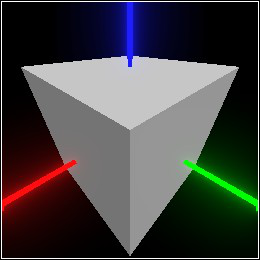These functions are helpful for writing algorithms that preprocess geometric data in a consistency-insensitive way.

#### 6.2Transformation Combiners and Constructors

 procedure(transform pict t) → Pict3D pict : Pict3D t : Affine
Transforms pict by applying t.

Examples:
 > (define pict (cube origin 1/2)) > (define t (affine-compose (move-z 1/4) (rotate-z 15))) > (transform pict t)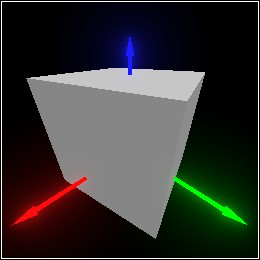procedure(move pict dv) → Pict3D pict : Pict3D dv : Dir
 procedure(move-x pict dx) → Pict3D pict : Pict3D dx : Real
 procedure(move-y pict dy) → Pict3D pict : Pict3D dy : Real
 procedure(move-z pict dz) → Pict3D pict : Pict3D dz : Real
Move pict in direction dv, or along an axis dx, dy or dz units.

 procedure(move dv) → Affine dv : Dir
 procedure(move-x dx) → Affine dx : Real
 procedure(move-y dy) → Affine dy : Real
 procedure(move-z dz) → Affine dz : Real
Transformation-returning versions of the above. Any (move pict ...) is equivalent to (transform pict (move ...)).

 procedure(scale pict dv) → Pict3D pict : Pict3D dv : (U Real Dir)
 procedure(scale-x pict dx) → Pict3D pict : Pict3D dx : Real
 procedure(scale-y pict dy) → Pict3D pict : Pict3D dy : Real
 procedure(scale-z pict dz) → Pict3D pict : Pict3D dz : Real
Scales pict by dv, or by dx, dy or dz units along an axis. If dv is a real number d, it’s equivalent to (dir d d d) (i.e. uniform scaling).

The center of scaling—i.e. the only point that does not move—is the origin. This is often not what you want. To scale pict with a different center, see scale/center.

 procedure(scale dv) → Linear dv : (U Real Dir)
 procedure(scale-x dx) → Linear dx : Real
 procedure(scale-y dy) → Linear dy : Real
 procedure(scale-z dz) → Linear dz : Real
Transformation-returning versions of the above. Any (scale pict ...) is equivalent to (transform pict (scale ...)).

 procedure(rotate pict axis angle) → Pict3D pict : Pict3D axis : Dir angle : Real
 procedure(rotate-x pict angle) → Pict3D pict : Pict3D angle : Real
 procedure(rotate-y pict angle) → Pict3D pict : Pict3D angle : Real
 procedure(rotate-z pict angle) → Pict3D pict : Pict3D angle : Real
Rotate pict, angle degrees counterclockwise around axis, or around the +x, +y or +z axis.

The center of rotation is the origin. This is often not what you want. To rotate pict around a different center, see rotate/center.

 procedure(rotate axis angle) → Linear axis : Dir angle : Real
 procedure(rotate-x angle) → Linear angle : Real
 procedure(rotate-y angle) → Linear angle : Real
 procedure(rotate-z angle) → Linear angle : Real
Transformation-returning versions of the above. Any (rotate pict ...) is equivalent to (transform pict (rotate ...)).

 procedure(scale/center pict dv [v]) → Pict3D pict : Pict3D dv : (U Real Dir) v : Pos = (center pict)
 procedure(scale-x/center pict dx [v]) → Pict3D pict : Pict3D dx : Real v : Pos = (center pict)
 procedure(scale-y/center pict dy [v]) → Pict3D pict : Pict3D dy : Real v : Pos = (center pict)
 procedure(scale-z/center pict dz [v]) → Pict3D pict : Pict3D dz : Real v : Pos = (center pict)
Scales pict by dv, or by dx, dy or dz units along an axis, with center v.

By default, v is the center of pict’s bounding box. If pict doesn’t have a bounding box (for example, it’s the empty-pict3d), the origin is the default center.

(scale/center pict dv v) is implemented as

(local-transform pict (scale dv) (move (pos- v origin)))

In other words, pict is scaled in the local coordinate space of an axis-aligned basis with center v.

 procedure(rotate/center pict axis angle [v]) → Pict3D pict : Pict3D axis : Dir angle : Real v : Pos = (center pict)
 procedure(rotate-x/center pict angle [v]) → Pict3D pict : Pict3D angle : Real v : Pos = (center pict)
 procedure(rotate-y/center pict angle [v]) → Pict3D pict : Pict3D angle : Real v : Pos = (center pict)
 procedure(rotate-z/center pict angle [v]) → Pict3D pict : Pict3D angle : Real v : Pos = (center pict)
Rotate pict, angle degrees counterclockwise around axis, or around the +x, +y or +z axis, with center v.

By default, v is the center of pict’s bounding box. If pict doesn’t have a bounding box (for example, it’s the empty-pict3d), the origin is the default center.

(rotate/center pict axis angle v) is implemented as

(local-transform pict (rotate axis angle) (move (pos- v origin)))

In other words, pict is rotated in the local coordinate space of an axis-aligned basis with center v.

procedure

 (point-at v dv [ #:angle angle #:up up #:normalize? normalize?]) → Affine
v : Pos
dv : Dir
angle : Real = 0
up : Dir = +z
normalize? : Any = #t
 (point-at v1 v2 [ #:angle angle #:up up #:normalize? normalize?]) → Affine
v1 : Pos
v2 : Pos
angle : Real = 0
up : Dir = +z
normalize? : Any = #t
Returns a transformation that “points” from v in direction dv, or from v1 to v2.

More specifically, the z axis of the transformation points as described. If normalize? isn’t #f, the z axis has distance 1. Otherwise, dv or (pos- v2 v1) is used as the z axis directly.

The other axes always have distance 1, are perpendicular to the z axis and each other, and are rotated about the z axis angle degrees counterclockwise (viewing the z axis head-on). When angle is 0, the y axis points opposite up (i.e. downward), and the x axis points rightward. When the z axis is parallel to up, the rotation is arbitrary but always defined.

This function is really more intuitive than the above discription might suggest. It’s best used to place basis groups and cameras, and to stretch shapes between two points (see relocate).

 procedure(relocate t1 t2) → Affine t1 : Affine t2 : Affine (relocate pict t1 t2) → Pict3D pict : Pict3D t1 : Affine t2 : Affine
Transforms pict from the local coordinate space defined by t1 into the local coordinate space defined by t2, or returns an Affine that does so.

For example, suppose we define a cylinder centered on the origin, and we want to stretch it between two arbitrary points.
 > (define pict (with-color (rgba "red" 0.9) (cylinder origin (dir 1/4 1/4 1/2))))
> (define pict-t (point-at (pos 0 0 -1/2) +z))
 > (combine pict (basis 'pict-t pict-t))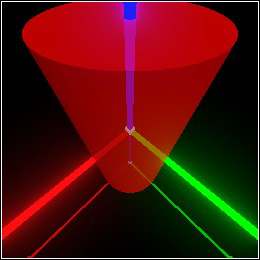(We’re only using basis to visualize the transformation pict-t.) To stretch it between v1 and v2, we define a point-at transformation:
> (define v1 (pos 1 0 1))
> (define v2 (pos 0 1 1))
> (define new-t (point-at v1 v2 #:normalize? #f))
 > (combine (sphere v1 0.2) (sphere v2 0.2) (basis 'new-t new-t))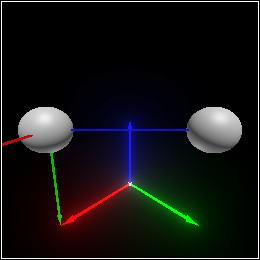Then we can use relocate to move pict into the new coordinate space:
 > (combine (sphere v1 0.2) (sphere v2 0.2) (relocate pict pict-t new-t))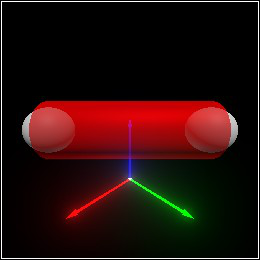(relocate t1 t2) is implemented as
In other words, undo transformation t1, then do transformation t2.

 procedure(local-transform t local-t) → Affine t : Affine local-t : Affine (local-transform pict t local-t) → Pict3D pict : Pict3D t : Affine local-t : Affine
Applies t to pict in the local coordinate space defined by local-t, or returns an Affine that does so.

(local-transform t local-t) is implemented as

(affine-compose local-t (relocate local-t t))

which is equivalent to

(affine-compose local-t t (affine-inverse local-t))

This operation is also known as a change of basis.

The scale/center and rotate/center functions are defined using local-transform, to apply scaling and rotating transformations in a local coordinate space with a center other than the origin.

 procedure(transform-pos v t) → Pos v : Pos t : Affine
 procedure(transform-dir dv t) → Dir dv : Dir t : Affine
 procedure(transform-norm dv t) → Dir dv : Dir t : Affine
Apply affine transformation t to a position, direction or normal.

The difference between applying a transformation to a direction and to a normal is best communicated with an extended example.

Examples:
> (define pict (sphere origin 1))
 > (define-values (vs dvs) (for*/lists (vs dvs) ([dx  (in-range -1 5/4 1/4)] [dy  (in-range -1 5/4 1/4)]) (define data (surface/data pict (dir dx dy 1))) (values (surface-data-pos data) (surface-data-normal data))))
 > (combine pict (for/list ([v  (in-list vs)] [dv  (in-list dvs)]) (arrow v (dir-scale dv 0.5))))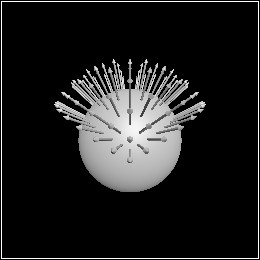> (define t (scale-z 1/4))
 > (combine (transform pict t) (for/list ([v  (in-list vs)] [dv  (in-list dvs)]) (arrow (transform-pos v t) (dir-scale (transform-dir dv t) 0.5))))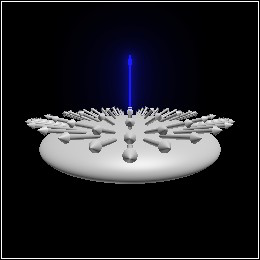> (combine (transform pict t) (for/list ([v  (in-list vs)] [dv  (in-list dvs)]) (arrow (transform-pos v t) (dir-scale (transform-norm dv t) 0.5))))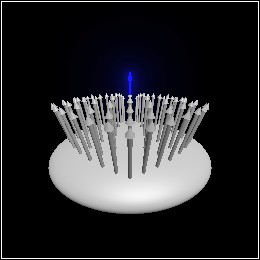In the second Pict3D, the directions have been flattened along with the sphere. In the third, they maintain their orthogonality to the surface.

 procedure(camera-transform pict) → (U #f Affine) pict : Pict3D
Returns the camera used to orient the initial view, if at least one 'camera basis is in pict. If there are none, returns #f.

procedure

 (camera-ray-dir t [ #:width width #:height height #:z-near z-near #:z-far z-far #:fov fov]) → (-> Real Real Dir)
t : Affine
width : Integer = (current-pict3d-width)
height : Integer = (current-pict3d-height)
z-near : Real = (current-pict3d-z-near)
z-far : Real = (current-pict3d-z-far)
fov : Real = (current-pict3d-fov)
Creates a function that accepts screen coordinates and returns the direction of the ray from the camera’s origin to those coordinates in the camera’s local coordinate space.

If (<= 0 x width) and (<= 0 y height), then the position (pos+ (affine-origin t) ((camera-ray-dir t) x y)) lies on the z-near plane.

A common use of camera-ray-dir is to find objects under a mouse cursor in a rendered Pict3D.

A less common use is to build a ray tracer in very few lines of code, such as the following.

 > (define p (combine (cube origin 1/2) (for*/list ([i  (in-range -1 2)] [j  (in-range -1 2)] [k  (in-range -1 2)]) (sphere (pos (* 0.35 i) (* 0.35 j) (* 0.35 k)) 0.2))))
> p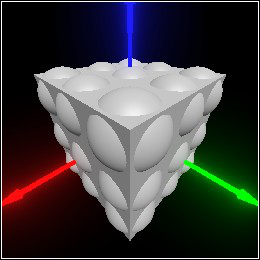> (define t ((current-pict3d-auto-camera) p))
> (define v0 (affine-origin t))
> (define ray-dir (camera-ray-dir t))
> (define l (pos 1.0 1.5 2.0))
> (require images/flomap)
 > (flomap->bitmap (build-flomap 1 (current-pict3d-width) (current-pict3d-height) ; for each screen coordinate on a grayscale surface... (λ (_ x y) ; trace from the camera origin through the screen at x,y (define-values (v n) (trace/normal p v0 (ray-dir (+ x 0.5) (+ y 0.5)))) (cond [(and v n) ; direction from surface point to light source (define dl (pos- l v)) ; distance squared to light source (define m^2 (dir-dist^2 dl)) ; 3.0 brightness, inverse-square attenuation (define a (/ 3.0 m^2)) ; Lambert's cosine law (fully diffuse material) (define b (max 0.0 (/ (dir-dot n dl) (sqrt m^2)))) ; compute brightness, apply gamma correction (expt (* a b) (/ 1.0 2.2))] [else  0.0]))))